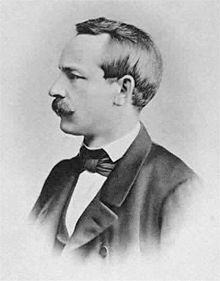## Monday 25 March 2019

### The Christoffel SymbolChristoffel
At last we move on to chapter three on curvature and immediately we find the Christoffel symbol Γ which is upper case γ. Elwin Bruno Christoffel was a mathematician, born in Prussia in 1829, and he studied at the University of Berlin. "He introduced fundamental concepts of differential geometry, opening the way for the development of tensor calculus, which would later provide the mathematical basis for general relativity."

I followed equations (3.5)-(3.10) carefully because I fell into the same tramp as I had before on one step and found an error of a sign in Carroll's  (3.10) which is the important equation for the transformation of the connection. This is fairly obvious because it comes from (3.9) and a + term has gone to the other side of the equation without changing sign. It was also confirmed by notes I found at  Physics 171 and the proof about Carroll's (3.26), see  below. I struggled with that proof (and part 2 of exercise 1) for too long. Eventually I found it after I found another erroneous proof on another website which nevertheless gave me a great new indexing trick (note d). I have corrected the error here.

I still do not understand Carroll's third rule for covariant derivatives that they commute with contractions  but he never seems to use it. Its meaning  provoked a discussion on physics forums which did not help me. In another discussion my false assumption about commuting partial derivatives was exposed.

The three most important equations here are$${\mathrm{\Gamma }}^{\nu '}_{\mu '\lambda '}=\frac{\partial x^{\mu }}{\partial x^{\mu '}}\frac{\partial x^{\lambda }}{\partial x^{\lambda '}}\frac{\partial x^{\nu '}}{\partial x^{\nu }}{\mathrm{\Gamma }}^{\nu }_{\mu \lambda }-\frac{\partial x^{\mu }}{\partial x^{\mu '}}\frac{\partial x^{\lambda }}{\partial x^{\lambda '}}\frac{{\partial }^2x^{\nu '}}{\partial x^{\mu }\partial x^{\lambda }}$$ and $${\mathrm{\Gamma }}^{\nu '}_{\mu '\lambda '}=\frac{\partial x^{\mu }}{\partial x^{\mu '}}\frac{\partial x^{\lambda }}{\partial x^{\lambda '}}\frac{\partial x^{\nu '}}{\partial x^{\nu }}{\mathrm{\Gamma }}^{\nu }_{\mu \lambda }+\frac{\partial x^{\nu '}}{\partial x^{\lambda }}\frac{{\partial }^2x^{\lambda }}{\partial x^{\mu '}\partial x^{\lambda '}}$$ which are alternatives for the transformation of a connection. The first one was Carroll's (3.10) (corrected.)

The third is Carroll's (3.27). He writes it is "one of the most important equations in this subject; commit it to memory." It is for a torsion-free (${\mathrm{\Gamma }}^{\lambda }_{\mu \nu }={\mathrm{\Gamma }}^{\lambda }_{\nu \mu }$) metric-compatible (${\mathrm{\nabla }}_{\rho }g_{\mu \nu }=0$) connection and is$${\mathrm{\Gamma }}^{\sigma }_{\mu \nu }=\frac{1}{2}g^{\sigma \rho }\left({\partial }_{\mu }g_{\nu \rho }+{\partial }_{\nu }g_{\rho \mu }-{\partial }_{\rho }g_{\mu \nu }\right)$$For some reason Carroll writes ${\mathrm{\Gamma }}^{\lambda }_{\mu \nu }={\mathrm{\Gamma }}^{\lambda }_{\nu \mu }$ as ${\mathrm{\Gamma }}^{\lambda }_{\mu \nu }={\mathrm{\Gamma }}^{\lambda }_{(\mu \nu )}$ which is the same but more complicated. The brackets are the symmetrisation operator.

1.2.